CLAT  >  Percentage And Its Applications

# Percentage And Its Applications | Quantitative Techniques for CLAT

 1 Crore+ students have signed up on EduRev. Have you?

‘Per cent’ means ‘out of one hundred’. Symbol % is used to denote per cent

Some examples to convert a per cent into a fraction, ratio or decimal and vice versa.1.
(i) 50% = 0.50

(ii) 7% = 0.07

(iii) 90% =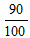= 0.90

(iv) 1% = 0.01

2.
(i) 10% of 100 = 10

(ii) 20% of 500 = 100

(iii) 30% of 1000 = 300

3.
(i) 5 out of 25 = 20%

(ii) Re 1 out of Rs 25 = 4%

(iii) 10 out of 50 = 20%

Example 1.

(a) If 25% of X= 30. find X

Sol. 25% of X is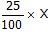and it is equal to 30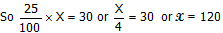(b) If 24% of X is 48, then find X

Sol. 24% of X is 48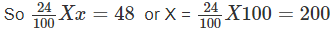Example 2: Vijay deposits Rs 45 per month in the bank. If this amount is 15% of his monthly income, find his monthly income.

Solution: Let his monthly income be Rs X

As per the given statement 15% of X is 45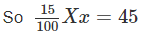or x =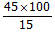= 300 RS

Example 3: Gunpowder contains 75% nitre and 10% sulphur. The rest of the material is charcoal. Find the amount of each of the contents in 9 kg of gunpowder.

Solution: Weight of gunpowder = 9 kg

Contents are – nitre = 75%, Sulphur = 10% charcoal = 100-75-10 = 15%

Nitre in 9 kg =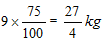= 6.75 kg

Sulphur in 9 kg =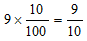= 0.90 kg

Charcoal in 9 kg =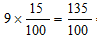= 1.35 kg

Question for Percentage And Its Applications
Try yourself:If 4.6% of X is 23 find X

Example 4: Ankits salary is 25% more than that of Anil. What percent is Anil’s salary less than Ankits?

Solution: Let Anil’s salary be Rs 100

Then Ankit gets 25% more than Anil = 125

If Ankit’s salary is Rs 125 Anil’s = 100

If Ankit’s salary is Rs 100 then Anil’s =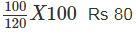So, Anils salary is Rs 80 when Ankit’s is Rs 100

So, Anil salary is 20% less than Ankit

Example 5: Raman loses 20% of his money. After spending 25% of the remaining, he has Rs 480. How much did he originally had?

Solution: Suppose Raman had Rs 100 in the beginning. He lost 20% i.e. Rs. 20

Remainder = Rs 100 – Rs 20 = Rs 80

Out of Rs 80 he spent 25%, which is equal to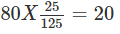Remainder = Rs 80 – Rs 20 = Rs 60

If remainder is Rs 60, he originally had = Rs 100

If remainder is Rs1, he originally had =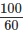If remainder is Rs 480 he originally had =Question for Percentage And Its Applications
Try yourself:Example 6: The salary of a person has been increased by 25%. By what per cent should the salary be reduced so that he gets the original salary. (answer in interger only)

Example 6: The price of a shirt in Dec 1997 was Rs 250. In Jan. 1998 it was increased by 10% and in Oct 1998 it was reduced by 10%. What in the new price of the shirt.

Solution: Price in Dec 1997 = Rs 250

Price in Jan 1998 =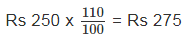Price in Oct 1998 = 275 x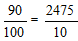= Rs 247.50

Question for Percentage And Its Applications
Try yourself:
Example 12: When 75% of a number is added to 75, the result is the same number. Find the number. (answer in interger only)

The document Percentage And Its Applications | Quantitative Techniques for CLAT is a part of the CLAT Course Quantitative Techniques for CLAT.
All you need of CLAT at this link: CLAT

## Quantitative Techniques for CLAT

56 videos|35 docs|91 tests

## Quantitative Techniques for CLAT

56 videos|35 docs|91 tests

Track your progress, build streaks, highlight & save important lessons and more!

,

,

,

,

,

,

,

,

,

,

,

,

,

,

,

,

,

,

,

,

,

;